×#### Thank you for registering.

One of our academic counsellors will contact you within 1 working day.

Click to Chat

1800-1023-196

+91-120-4616500

CART 0

• 0

MY CART (5)

Use Coupon: CART20 and get 20% off on all online Study Material

ITEM
DETAILS
MRP
DISCOUNT
FINAL PRICE
Total Price: Rs.

There are no items in this cart.
Continue Shopping• Complete JEE Main/Advanced Course and Test Series
• OFFERED PRICE: Rs. 15,900
• View Details

```Chapter 13: Complex Numbers – Exercise 13.1

Complex Numbers – Exercise 13.1 – Q.1(i)

We know that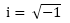i2 = – 1

i3 = – i

i4  = 1

In order to find in where n > 4, we divide n by 4 to get quotient p and remainder q, so that

n = 4p + q, o ≤ q < 4

Then in = i4o+q

= i4o ×iq

= (i4)q × iq

= ip × iq

= iq             [∵ 1p-1]

Hence in = iq, where o ≤ q < 4

∴  i457 = i4×114 × i1

= i1

= i

Complex Numbers – Exercise 13.1 – Q.1(ii)

We know thati2 =  – 1

i3 =  – i

i4 = 1

In order to find in where n > 4, we divide n by 4 to get quotient p and remainder q, so that

n = 4p + q, o ≤ q < 4

Then in = i4o+q

= i4o × iq

= (i4)p ×  iq

= 1p × iq

= iq          [∵ 1p-1]

Hence in = iq, where o ≤ q < 4

∴ i528 = i4×132

= (i4)132

= 1132

= 1

∴  (i528) = 1

Complex Numbers – Exercise 13.1 – Q.1(iii)

We know that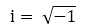i2 = – 1

i3 = – i

i4 = 1

In order to find in where n > 4, we divide n by 4 to get quotient p and remainder q, so that

n = 4p + q, o ≤ q < 4

Then in = i4o+q

= i4o × iq

= (i4)p ×  iq

= 1p × iq

= iq            [∵  1p-1]

Hence in = iq, where o ≤ q < 4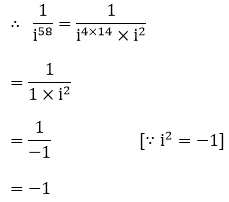Complex Numbers – Exercise 13.1 – Q.1(iv)

We know that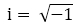i2 = – 1

i3 = – i

i4 = 1

In order to find in where n > 4, we divide n by 4 to get quotient p and remainder q, so that

n = 4p + q, o ≤ q < 4

Then in = i4o+q

= i4o × iq

= (i4)p ×  iq

= 1p × iq

= iq            [∵ 1p-1]

Hence in = iq, where o ≤ q < 4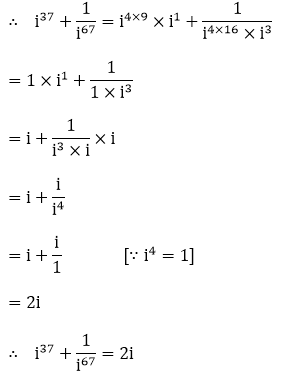Complex Numbers – Exercise 13.1 – Q.1(v)

We know that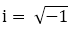i2 = – 1

i3 = – i

i4 = 1

In order to find in where n > 4, we divide n by 4 to get quotient p and remainder q, so that

n = 4p + q, o ≤ q < 4

Then in = i4o+q

= i4o × iq

= (i4)p ×  iq

= 1p × iq

= iq                   [∵ 1p-1]

Hence in = iq, where o ≤ q < 4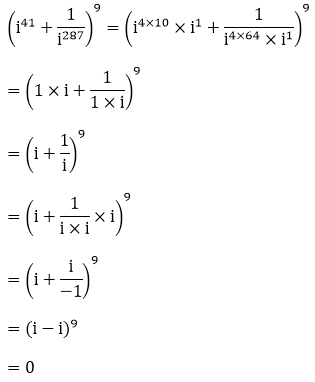Complex Numbers – Exercise 13.1 – Q.1(vi)

We know thati2 = – 1

i3 = – i

i4 = 1

In order to find in where n > 4, we divide n by 4 to get quotient p and remainder q, so that

n = 4p + q, o ≤ q < 4

Then in = i4o+q

= i4o × iq

= (i4)p ×  iq

= 1p × iq

= iq                   [∵ 1p-1]

Hence in = iq, where o ≤ q < 4

(i77 + i70 + i87 + i414)3 = (i4×19 × i1 + i4×17 × i2 + i4×21 × i3 + i4×103 × i2)3

= (1 × i + 1 × i2 + 1 × i3 + 1 × i2)3

= (i – 1 –  i –1)3

= ( – 2)3

= – 8

∴  (i77 + i70 + i87 + i414)3 = – 8

Complex Numbers – Exercise 13.1 – Q.1(vii)

We know that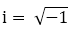i2 =  – 1

i3 = – i

i4 = 1

In order to find in where n > 4, we divide n by 4 to get quotient p and remainder q, so that

n = 4p + q, o ≤ q < 4

Then in = i4o+q

= i4o × iq

= (i4)p ×  iq

= 1p × iq

= iq                   [∵ 1p-1]

Hence in = iq, where o ≤ q < 4

∴  i30 + i40 + i60 = i4×7 ×i2 + i4×10 + i4×15

= 1 × i2 + 1 + 1

= – 1 + 1 + 1

= 1

∴  i30 + i40 + i60 = 1

Complex Numbers – Exercise 13.1 – Q.1(viii)

We know that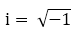i2 = – 1

i3 = – i

i4 = 1

In order to find in where n > 4, we divide n by 4 to get quotient p and remainder q, so that

n = 4p + q, o ≤ q < 4

Then in = i4o+q

= i4o × iq

= (i4)p ×  iq

= 1p × iq

= iq          [∵ 1p-1]

Hence in = iq, where o ≤ q < 4

i49 + i68 + i89 + i110 = i4×12 × i1 + i4×17 + i4×22 × i1 + i4×27 × i2

= 1 × i + 1 + 1 × i + 1 × i2

= i + 1 + i - 1

= 2i

∴ i49 + i68 + i89 + i110 = 2i

Complex Numbers – Exercise 13.1 – Q.2

1 + i10  +  i20 + i30 = 1 + i4×2 × i2 + i4×5 + i4×7 × i2

= 1 + 1 × i2 + 1 + 1 × i2

= 1 - 1 + 1 - 1

=  0, which is real number
```### Course Features

• 728 Video Lectures
• Revision Notes
• Previous Year Papers
• Mind Map
• Study Planner
• NCERT Solutions
• Discussion Forum
• Test paper with Video Solution